This is a fixed-text formatted version of a Jupyter notebook

# Fermi-LAT data with Gammapy¶

## Introduction¶

This tutorial will show you how to work with Fermi-LAT data with Gammapy. As an example, we will look at the Galactic center region using the high-energy dataset that was used for the 3FHL catalog, in the energy range 10 GeV to 2 TeV.

We note that support for Fermi-LAT data analysis in Gammapy is very limited. For most tasks, we recommend you use Fermipy, which is based on the Fermi Science Tools (Fermi ST).

Using Gammapy with Fermi-LAT data could be an option for you if you want to do an analysis that is not easily possible with Fermipy and the Fermi Science Tools. For example a joint likelihood fit of Fermi-LAT data with data e.g. from H.E.S.S., MAGIC, VERITAS or some other instrument, or analysis of Fermi-LAT data with a complex spatial or spectral model that is not available in Fermipy or the Fermi ST.

Besides Gammapy, you might want to look at are Sherpa or 3ML. Or just using Python to roll your own analyis using several existing analysis packages. E.g. it it possible to use Fermipy and the Fermi ST to evaluate the likelihood on Fermi-LAT data, and Gammapy to evaluate it e.g. for IACT data, and to do a joint likelihood fit using e.g. iminuit or emcee.

To use Fermi-LAT data with Gammapy, you first have to use the Fermi ST to prepare an event list (using gtselect and gtmktime, exposure cube (using gtexpcube2 and PSF (using gtpsf). You can then use gammapy.data.EventList, gammapy.maps and the gammapy.irf.EnergyDependentTablePSF to read the Fermi-LAT maps and PSF, i.e. support for these high-level analysis products from the Fermi ST is built in. To do a 3D map analyis, you can use Fit for Fermi-LAT data in the same way that it’s use for IACT data. This is illustrated in this notebook. A 1D region-based spectral analysis is also possible, this will be illustrated in a future tutorial.

## Setup¶

IMPORTANT: For this notebook you have to get the prepared 3fhl dataset provided in your $GAMMAPY_DATA. Note that the 3fhl dataset is high-energy only, ranging from 10 GeV to 2 TeV. :  # Check that you have the prepared Fermi-LAT dataset # We will use diffuse models from here !ls -1$GAMMAPY_DATA/fermi_3fhl

fermi_3fhl_events_selected.fits.gz
fermi_3fhl_exposure_cube_hpx.fits.gz
fermi_3fhl_psf_gc.fits.gz
gll_iem_v06_cutout.fits
iso_P8R2_SOURCE_V6_v06.txt

:

%matplotlib inline
import matplotlib.pyplot as plt

:

import numpy as np
from astropy import units as u
from astropy.coordinates import SkyCoord
from gammapy.data import EventList
from gammapy.irf import EnergyDependentTablePSF, EDispKernel
from gammapy.maps import Map, MapAxis, WcsNDMap, WcsGeom
from gammapy.modeling.models import (
PowerLawSpectralModel,
PointSpatialModel,
SkyModel,
SkyDiffuseCube,
Models,
create_fermi_isotropic_diffuse_model,
)
from gammapy.cube import MapDataset, PSFKernel
from gammapy.modeling import Fit


## Events¶

To load up the Fermi-LAT event list, use the gammapy.data.EventList class:

:

events = EventList.read(
"$GAMMAPY_DATA/fermi_3fhl/fermi_3fhl_events_selected.fits.gz" ) print(events)  EventList info: - Number of events: 697317 - Median energy: 1.59e+04 MeV  The event data is stored in a astropy.table.Table object. In case of the Fermi-LAT event list this contains all the additional information on positon, zenith angle, earth azimuth angle, event class, event type etc. :  events.table.colnames  :  ['ENERGY', 'RA', 'DEC', 'L', 'B', 'THETA', 'PHI', 'ZENITH_ANGLE', 'EARTH_AZIMUTH_ANGLE', 'TIME', 'EVENT_ID', 'RUN_ID', 'RECON_VERSION', 'CALIB_VERSION', 'EVENT_CLASS', 'EVENT_TYPE', 'CONVERSION_TYPE', 'LIVETIME', 'DIFRSP0', 'DIFRSP1', 'DIFRSP2', 'DIFRSP3', 'DIFRSP4']  :  events.table[:5][["ENERGY", "RA", "DEC"]]  :  Table length=5 ENERGYRADEC MeVdegdeg float32float32float32 12856.5205139.64438-9.93702 14773.319177.0445460.55275 23273.527110.2132537.002018 41866.125334.8528717.577398 42463.074316.8667648.152477 :  print(events.time.iso) print(events.time[-1].iso)  2008-08-04 15:49:26.782 2015-07-30 11:00:41.226  :  energy = events.energy energy.info("stats")  mean = 28905.451171875 MeV std = 61051.7421875 MeV min = 10000.03125 MeV max = 1998482.75 MeV n_bad = 0 length = 697317  As a short analysis example we will count the number of events above a certain minimum energy: :  for e_min in [10, 100, 1000] * u.GeV: n = (events.energy > e_min).sum() print(f"Events above {e_min:4.0f}: {n:5.0f}")  Events above 10 GeV: 697317 Events above 100 GeV: 23628 Events above 1000 GeV: 544  ## Counts¶ Let us start to prepare things for an 3D map analysis of the Galactic center region with Gammapy. The first thing we do is to define the map geometry. We chose a TAN projection centered on position (glon, glat) = (0, 0) with pixel size 0.1 deg, and four energy bins. :  gc_pos = SkyCoord(0, 0, unit="deg", frame="galactic") energy_axis = MapAxis.from_edges( [10, 30, 100, 300, 2000], name="energy", unit="GeV", interp="log" ) counts = Map.create( skydir=gc_pos, npix=(100, 80), proj="TAN", frame="galactic", binsz=0.1, axes=[energy_axis], dtype=float, ) # We put this call into the same Jupyter cell as the Map.create # because otherwise we could accidentally fill the counts # multiple times when executing the fill_by_coord multiple times. counts.fill_by_coord({"skycoord": events.radec, "energy": events.energy})  :  counts.geom.axes  :  MapAxis name : energy unit : 'GeV' nbins : 4 node type : edges edges min : 1.0e+01 GeV edges max : 2.0e+03 GeV interp : log  :  counts.sum_over_axes().smooth(2).plot(stretch="sqrt", vmax=30);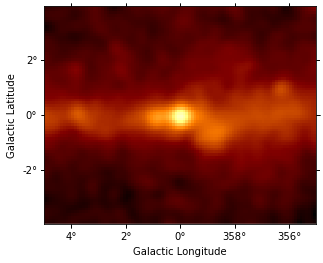## Exposure¶ The Fermi-LAT datatset contains the energy-dependent exposure for the whole sky as a HEALPix map computed with gtexpcube2. This format is supported by gammapy.maps directly. Interpolating the exposure cube from the Fermi ST to get an exposure cube matching the spatial geometry and energy axis defined above with Gammapy is easy. The only point to watch out for is how exactly you want the energy axis and binning handled. Below we just use the default behaviour, which is linear interpolation in energy on the original exposure cube. Probably log interpolation would be better, but it doesn’t matter much here, because the energy binning is fine. Finally, we just copy the counts map geometry, which contains an energy axis with node_type="edges". This is non-ideal for exposure cubes, but again, acceptable because exposure doesn’t vary much from bin to bin, so the exact way interpolation occurs in later use of that exposure cube doesn’t matter a lot. Of course you could define any energy axis for your exposure cube that you like. :  exposure_hpx = Map.read( "$GAMMAPY_DATA/fermi_3fhl/fermi_3fhl_exposure_cube_hpx.fits.gz"
)
# Unit is not stored in the file, set it manually
exposure_hpx.unit = "cm2 s"
print(exposure_hpx.geom)
print(exposure_hpx.geom.axes)

HpxGeom

axes       : ['skycoord', 'energy']
shape      : (49152, 18)
ndim       : 3
nside      : 64
nested     : False
frame   : icrs
projection : HPX
center     : 0.0 deg, 0.0 deg

MapAxis

name       : energy
unit       : 'MeV'
nbins      : 18
node type  : center
center min : 1.0e+04 MeV
center max : 2.0e+06 MeV
interp     : log


:

exposure_hpx.plot();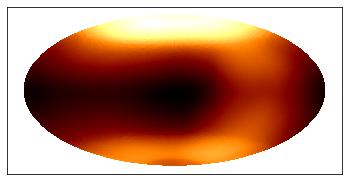:

# For exposure, we choose a geometry with node_type='center',
# whereas for counts it was node_type='edge'
axis = MapAxis.from_nodes(
counts.geom.axes.center, name="energy", unit="GeV", interp="log"
)
geom = WcsGeom(wcs=counts.geom.wcs, npix=counts.geom.npix, axes=[axis])

coord = counts.geom.get_coord()
data = exposure_hpx.interp_by_coord(coord)

:

exposure = WcsNDMap(geom, data, unit=exposure_hpx.unit, dtype=float)
print(exposure.geom)
print(exposure.geom.axes)

WcsGeom

axes       : ['lon', 'lat', 'energy']
shape      : (100, 80, 4)
ndim       : 3
frame   : galactic
projection : TAN
center     : 0.0 deg, 0.0 deg
width      : 10.0 deg x 8.0 deg

MapAxis

name       : energy
unit       : 'GeV'
nbins      : 4
node type  : center
center min : 1.7e+01 GeV
center max : 7.7e+02 GeV
interp     : log


:

# Exposure is almost constant accross the field of view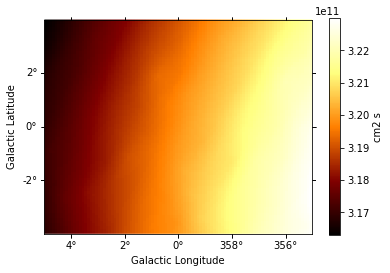:

# Exposure varies very little with energy at these high energies
energy = [10, 100, 1000] * u.GeV
exposure.get_by_coord({"skycoord": gc_pos, "energy": energy})

:

array([3.19917466e+11, 3.27536547e+11, 3.03032026e+11])


## Galactic diffuse background¶

The Fermi-LAT collaboration provides a galactic diffuse emission model, that can be used as a background model for Fermi-LAT source analysis.

Diffuse model maps are very large (100s of MB), so as an example here, we just load one that represents a small cutout for the Galactic center region.

:

diffuse_galactic_fermi = Map.read(
"$GAMMAPY_DATA/fermi_3fhl/gll_iem_v06_cutout.fits" ) # Unit is not stored in the file, set it manually diffuse_galactic_fermi.unit = "cm-2 s-1 MeV-1 sr-1" print(diffuse_galactic_fermi.geom) print(diffuse_galactic_fermi.geom.axes)  WcsGeom axes : ['lon', 'lat', 'energy'] shape : (88, 48, 30) ndim : 3 frame : galactic projection : CAR center : 0.0 deg, -0.1 deg width : 11.0 deg x 6.0 deg MapAxis name : energy unit : 'MeV' nbins : 30 node type : center center min : 5.8e+01 MeV center max : 5.1e+05 MeV interp : lin  WARNING: FITSFixedWarning: 'datfix' made the change 'Set DATE-REF to '1858-11-17' from MJD-REF'. [astropy.wcs.wcs]  :  # Interpolate the diffuse emission model onto the counts geometry # The resolution of diffuse_galactic_fermi is low: bin size = 0.5 deg # We use interp=3 which means cubic spline interpolation coord = counts.geom.get_coord() data = diffuse_galactic_fermi.interp_by_coord( {"skycoord": coord.skycoord, "energy": coord["energy"]}, interp=3 ) diffuse_galactic = WcsNDMap( exposure.geom, data, unit=diffuse_galactic_fermi.unit ) print(diffuse_galactic.geom) print(diffuse_galactic.geom.axes)  WcsGeom axes : ['lon', 'lat', 'energy'] shape : (100, 80, 4) ndim : 3 frame : galactic projection : TAN center : 0.0 deg, 0.0 deg width : 10.0 deg x 8.0 deg MapAxis name : energy unit : 'GeV' nbins : 4 node type : center center min : 1.7e+01 GeV center max : 7.7e+02 GeV interp : log  :  diffuse_gal = SkyDiffuseCube(diffuse_galactic)  :  diffuse_gal.map.slice_by_idx({"energy": 0}).plot(add_cbar=True);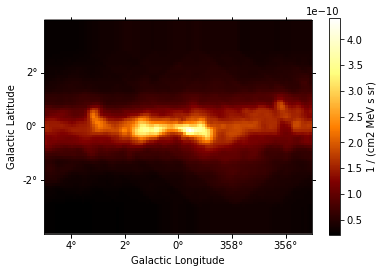:  # Exposure varies very little with energy at these high energies energy = np.logspace(1, 3, 10) * u.GeV dnde = diffuse_gal.map.interp_by_coord( {"skycoord": gc_pos, "energy": energy}, interp="linear", fill_value=None ) plt.plot(energy.value, dnde, "*") plt.loglog() plt.xlabel("Energy (GeV)") plt.ylabel("Flux (cm-2 s-1 MeV-1 sr-1)")  :  Text(0, 0.5, 'Flux (cm-2 s-1 MeV-1 sr-1)')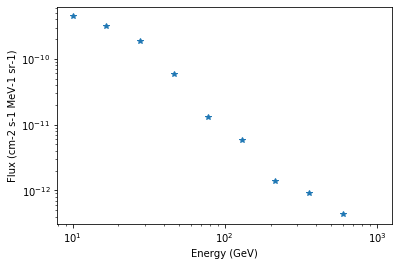:  # TODO: show how one can fix the extrapolate to high energy # by computing and padding an extra plane e.g. at 1e3 TeV # that corresponds to a linear extrapolation  ## Isotropic diffuse background¶ To load the isotropic diffuse model with Gammapy, use the gammapy.modeling.models.TemplateSpectralModel. We are using 'fill_value': 'extrapolate' to extrapolate the model above 500 GeV: :  filename = "$GAMMAPY_DATA/fermi_3fhl/iso_P8R2_SOURCE_V6_v06.txt"

diffuse_iso = create_fermi_isotropic_diffuse_model(
filename=filename, interp_kwargs={"fill_value": None}
)


We can plot the model in the energy range between 50 GeV and 2000 GeV:

:

erange = [50, 2000] * u.GeV
diffuse_iso.spectral_model.plot(erange, flux_unit="1 / (cm2 MeV s)");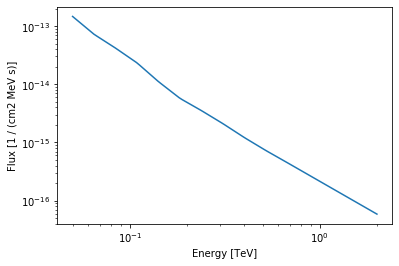## PSF¶

Next we will tke a look at the PSF. It was computed using gtpsf, in this case for the Galactic center position. Note that generally for Fermi-LAT, the PSF only varies little within a given regions of the sky, especially at high energies like what we have here. We use the gammapy.irf.EnergyDependentTablePSF class to load the PSF and use some of it’s methods to get some information about it.

:

psf = EnergyDependentTablePSF.read(
"\$GAMMAPY_DATA/fermi_3fhl/fermi_3fhl_psf_gc.fits.gz"
)
print(psf)

EnergyDependentTablePSF
-----------------------

Axis info:
rad            : size =   300, min =  0.000 deg, max =  9.933 deg
energy         : size =    17, min = 10.000 GeV, max = 2000.000 GeV

Containment info:
68.0% containment radius at  10 GeV: 0.16 deg
68.0% containment radius at 100 GeV: 0.10 deg
95.0% containment radius at  10 GeV: 0.71 deg
95.0% containment radius at 100 GeV: 0.43 deg



To get an idea of the size of the PSF we check how the containment radii of the Fermi-LAT PSF vari with energy and different containment fractions:

:

plt.figure(figsize=(8, 5))
psf.plot_containment_vs_energy(linewidth=2, fractions=[0.68, 0.95])
plt.xlim(50, 2000)
plt.show()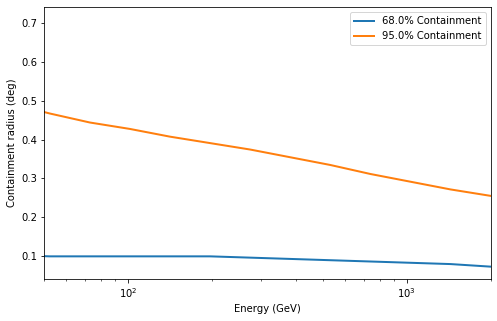In addition we can check how the actual shape of the PSF varies with energy and compare it against the mean PSF between 50 GeV and 2000 GeV:

:

plt.figure(figsize=(8, 5))

for energy in [100, 300, 1000] * u.GeV:
psf_at_energy = psf.table_psf_at_energy(energy)

erange = [50, 2000] * u.GeV
spectrum = PowerLawSpectralModel(index=2.3)
psf_mean = psf.table_psf_in_energy_band(energy_band=erange, spectrum=spectrum)

plt.xlim(1e-3, 0.3)
plt.ylim(1e3, 1e6)
plt.legend();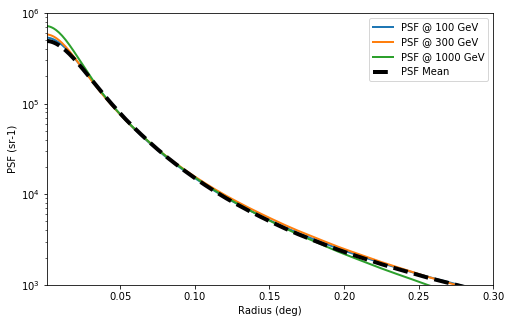:

# Let's compute a PSF kernel matching the pixel size of our map
psf_kernel = PSFKernel.from_table_psf(psf, counts.geom, max_radius="1 deg")

:

psf_kernel.psf_kernel_map.sum_over_axes().plot(stretch="log", add_cbar=True);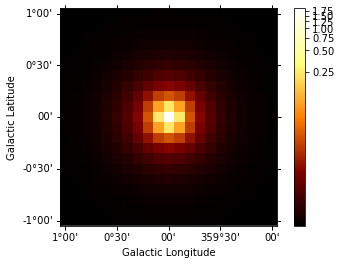### Energy Dispersion¶

For simplicity we assume a diagonal energy dispersion:

:

e_true = exposure.geom.axes.edges
e_reco = counts.geom.axes.edges
edisp = EDispKernel.from_diagonal_response(e_true=e_true, e_reco=e_reco)


## Fit¶

Finally, the big finale: let’s do a 3D map fit for the source at the Galactic center, to measure it’s position and spectrum. We keep the background normalization free.

:

spatial_model = PointSpatialModel(
lon_0="0 deg", lat_0="0 deg", frame="galactic"
)
spectral_model = PowerLawSpectralModel(
index=2.5, amplitude="1e-11 cm-2 s-1 TeV-1", reference="100 GeV"
)

source = SkyModel(spectral_model=spectral_model, spatial_model=spatial_model)

models = Models([source, diffuse_gal, diffuse_iso])

dataset = MapDataset(
models=models,
counts=counts,
exposure=exposure,
psf=psf_kernel,
edisp=edisp,
)

:

%%time
fit = Fit([dataset])
result = fit.run()

CPU times: user 10.1 s, sys: 239 ms, total: 10.3 s
Wall time: 10.5 s

:

print(result)

OptimizeResult

backend    : minuit
method     : minuit
success    : True
message    : Optimization terminated successfully.
nfev       : 467
total stat : 20050.89


:

dataset.parameters.to_table()

:

Table length=12
namevalueerrorunitminmaxfrozen
str9float64float64str14float64float64bool
lon_0-2.500e-02nandegnannanFalse
lat_0-3.979e-02nandeg-9.000e+019.000e+01False
index2.711e+00nannannanFalse
amplitude5.852e-10nancm-2 s-1 TeV-1nannanFalse
reference1.000e-01nanTeVnannanTrue
norm1.025e+00nannannanFalse
tilt0.000e+00nannannanTrue
reference1.000e+00nanTeVnannanTrue
value1.000e+00nansr-1nannanTrue
norm3.425e+00nannannanFalse
tilt0.000e+00nannannanTrue
reference1.000e+00nanTeVnannanTrue
:

residual = counts - dataset.npred()
residual.sum_over_axes().smooth("0.1 deg").plot(
);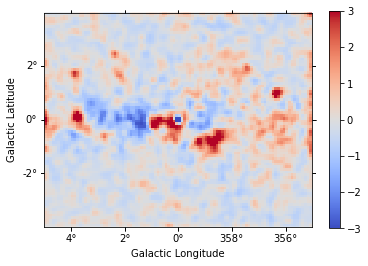## Exercises¶

• Fit the position and spectrum of the source SNR G0.9+0.1.

• Make maps and fit the position and spectrum of the Crab nebula.

## Summary¶

In this tutorial you have seen how to work with Fermi-LAT data with Gammapy. You have to use the Fermi ST to prepare the exposure cube and PSF, and then you can use Gammapy for any event or map analysis using the same methods that are used to analyse IACT data.

This works very well at high energies (here above 10 GeV), where the exposure and PSF is almost constant spatially and only varies a little with energy. It is not expected to give good results for low-energy data, where the Fermi-LAT PSF is very large. If you are interested to help us validate down to what energy Fermi-LAT analysis with Gammapy works well (e.g. by re-computing results from 3FHL or other published analysis results), or to extend the Gammapy capabilities (e.g. to work with energy-dependent multi-resolution maps and PSF), that would be very welcome!